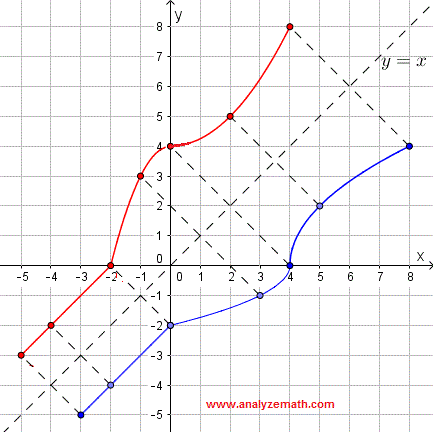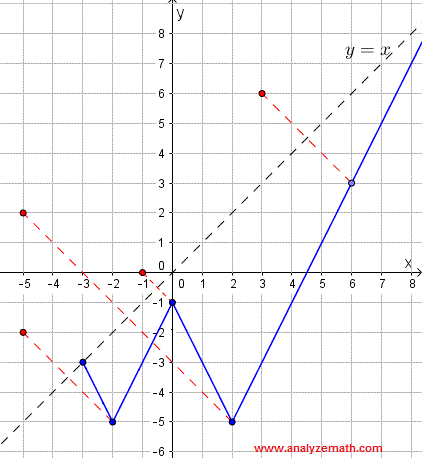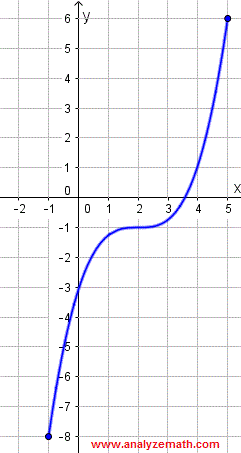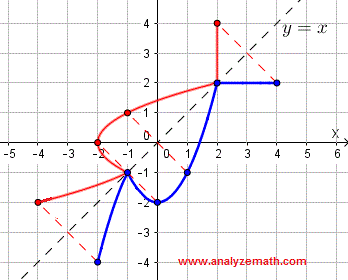# Find The Inverse of a Relation - Questions With Solutions

How to find the inverse of a relation given by its graph? Examples are presented along with detailed solutions and aslo questions with Solutions and explanations are included.

## Examples: How to find the inverse of a relation given by its graphs?

### Example 1

Sketch the graph of the inverse of the relation given by its graph below.Solution:
Step 1: Select points on the graph of the given relation and find their coordinates: blue points shown on graph below with the following coordinates.
(8,4) , (5,2) , (4,0) , (3,-1) , (0,-2) , (-2,-4) , (-3,-5)
Step 2: Reflect the points (by switching the x and y coordinates) obtained above on the line y = x : red points.
(4,8) , (2,5) , (0,4) , (-1,3) , (-2,0) , (-4,-2) , (-5,-3)Sketch the graph of the inverse of the given relation by connecting the inverted points as shown by the red graph below. The given graph and the inverse are reflection of each other on the line y = x.### Example 2

Sketch the graph of the inverse of the relation given by its graph below.Solution:
Step 1: Select and define points on the graph of the given relation by their coordinates as shown on graph below (blue points on the graph) .
(6,3) , (2,-5) , (0,-1) , (-2,-5) , (-3,-3)
Step 2: Reflect, on the line y = x, the points (by switching the x and y coordinates) obtained above as shown by the red points with coordinates.
(6,3) , (2,-5) , (0,-1) , (-2,-5) , (-3,-3)The graph of the inverse relation is obtained by connecting the inverted points as shown below so that the given graph and the inverse are reflection of each other on the line y = x.## Questions

Sketch the graph of the inverse of each of the relations given by its graph below:
a)b)## Solutions to the Above Questions

a) Solution to part a)
Step 1: Select points on the graph of the given relation and find their coordinates: blue points shown on graph below with the following coordinates.
(4,2) , (2,2) , (1,-1) , (0,-2) , (-1,-1) , (-2,-4)
Step 2: Reflect, on the line y = x, by switching the x and y coordinates of the points obtained above to obtain the red points.
(2,4) , (2,2) , (-1,1) , (-2,0) , (-1,-1) , (-4,-2)The inverse of the given relation is obtained by connecting the inverted points as shown by the red graph below. The given graph and the inverse are reflection of each other on the line y = x.b) Solution to part b)
Step 1: Select points on the graph of the given relation and find their coordinates: blue points shown on graph below with the following coordinates.
(5,6) , (4,1) , (2,-1) , (0,-3) , (-1,-8)
Step 2: Reflect the points (by switching the x and y coordinates) obtained above on the line y = x : red points.
(6,5) , (1,4) , (-1,2) , (-3,0) , (-8,-1)Sketch the graph of the inverse of the given relation by connecting the inverted points as shown by the red graph below so that the given graph and the inverse are reflection of each other on the line y = x.